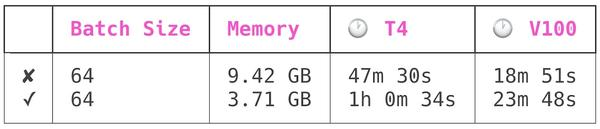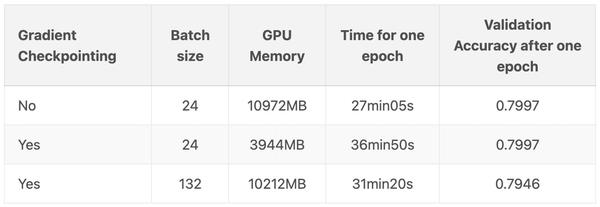• 问答
• 技术
• 实践
• 资源

## >>> 梯度检查点是如何起作用的

PyTorch 通过 torch.utils.checkpoint.checkpointtorch.utils.checkpoint.checkpoint_sequential 提供梯度检查点，根据官方文档的 notes，它实现了如下功能，在前向传播时，PyTorch 将保存模型中的每个函数的输入元组。在反向传播过程中，对于每个函数，输入元组和函数的组合以实时的方式重新计算，插入到每个需要它的函数的梯度公式中，然后丢弃。网络计算开销大致相当于每个样本通过模型前向传播开销的两倍。

## >>> 测试 API

PyTorch API 中有两个不同的梯度检查点方法，都在 torch.utils.checkpoint 命名空间中。两者中比较简单的一个是 checkpoint_sequential，它被限制用于顺序模型（例如使用 torch.nn.Sequential wrapper 的模型）。另一个是更灵活的 checkpoint，可以用于任何模块。

import torch
import torch.nn as nn

from torch.utils.checkpoint import checkpoint_sequential

# a trivial model
model = nn.Sequential(
nn.Linear(100, 50),
nn.ReLU(),
nn.Linear(50, 20),
nn.ReLU(),
nn.Linear(20, 5),
nn.ReLU()
)

# model input

# the number of segments to divide the model into
segments = 2

# finally, apply checkpointing to the model
# note the code that this replaces:
# out = model(input_var)
out = checkpoint_sequential(modules, segments, input_var)

# backpropagate
out.sum().backwards()

class CIFAR10Model(nn.Module):
def __init__(self):
super().__init__()
self.cnn_block_1 = nn.Sequential(*[
nn.ReLU(),
nn.ReLU(),
nn.MaxPool2d(kernel_size=2),
nn.Dropout(0.25)
])
self.cnn_block_2 = nn.Sequential(*[
nn.ReLU(),
nn.ReLU(),
nn.MaxPool2d(kernel_size=2),
nn.Dropout(0.25)
])
self.flatten = lambda inp: torch.flatten(inp, 1)
nn.Linear(64 * 8 * 8, 512),
nn.ReLU(),
nn.Dropout(0.5),
nn.Linear(512, 10)
])

def forward(self, X):
X = self.cnn_block_1(X)
X = self.cnn_block_2(X)
X = self.flatten(X)
return X

class CIFAR10Model(nn.Module):
def __init__(self):
super().__init__()
self.cnn_block_1 = nn.Sequential(*[
nn.ReLU(),
nn.ReLU(),
nn.MaxPool2d(kernel_size=2)
])
self.dropout_1 = nn.Dropout(0.25)
self.cnn_block_2 = nn.Sequential(*[
nn.ReLU(),
nn.ReLU(),
nn.MaxPool2d(kernel_size=2)
])
self.dropout_2 = nn.Dropout(0.25)
self.flatten = lambda inp: torch.flatten(inp, 1)
self.linearize = nn.Sequential(*[
nn.Linear(64 * 8 * 8, 512),
nn.ReLU()
])
self.dropout_3 = nn.Dropout(0.5)
self.out = nn.Linear(512, 10)

def forward(self, X):
X = self.cnn_block_1(X)
X = self.dropout_1(X)
X = checkpoint(self.cnn_block_2, X)
X = self.dropout_2(X)
X = self.flatten(X)
X = self.linearize(X)
X = self.dropout_3(X)
X = self.out(X)
return X

## >>> 基准测试

# code from model_5.py

cfg = transformers.PretrainedConfig.get_config_dict("bert-base-uncased")
cfg["output_hidden_states"] = True
cfg = transformers.BertConfig.from_dict(cfg)
self.bert = transformers.BertModel.from_pretrained(
"bert-base-uncased", config=cfg
)•0
•0
•86收藏

• 49

关注
• 127

获赞
• 7

精选文章

• 目标跟踪

• 目标跟踪分享

• 44
• 84
• 721
• 69
• 170